# Decimal Chart - Definition with Examples

The Complete K-5 Math Learning Program Built for Your Child

• 40 Million Kids

Loved by kids and parent worldwide

• 50,000 Schools

Trusted by teachers across schools

• Comprehensive Curriculum

Aligned to Common Core

## Decimal Chart

Place Value Chart

In a place value chart, as we move from right to left, the value of each place increases by 10 times.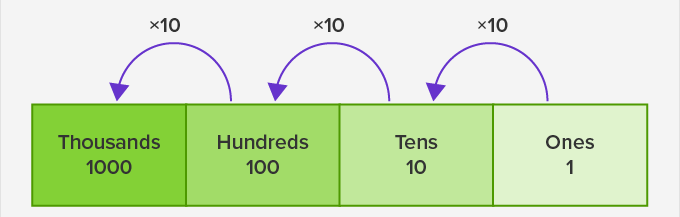And as we move from left to right, the value of each place decreases by one-tenth.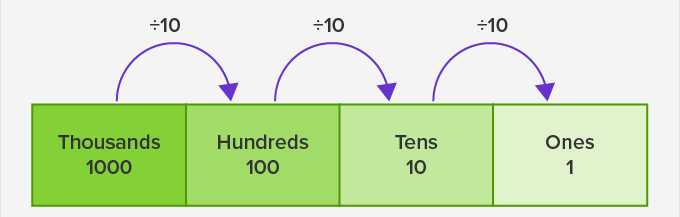On moving to the right of ones we get a place ten times smaller than 1, that is, one-tenth or ‘tenths’, which is a fractional part. Hence, we separate it by a decimal point. So, our place value chart looks like this: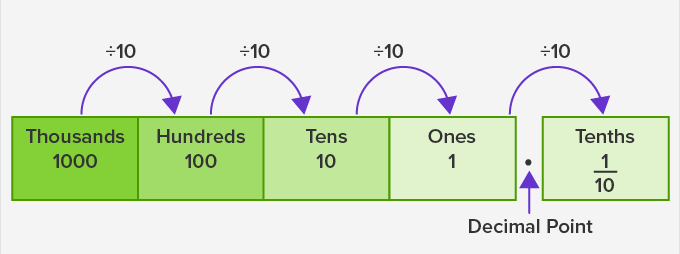The place to the right of ‘tenths’ is the ‘hundredths’ and the place to the right of ‘hundredths’ is the ‘thousandths’.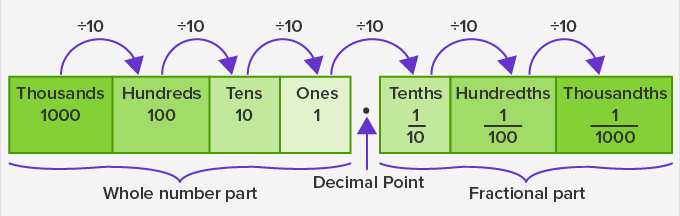Now we have our decimal place value chart ready. A decimal place value chart helps us to find the place value of the digits in a decimal number.

Example: Write the place value of the digits 3 and 6 in the number 4342.761

First, write the number in a decimal place value chart.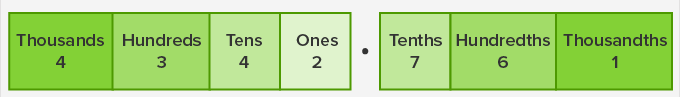Then, look at the place of the digit and find its place value.

The digit 3 is in the hundreds place. Therefore, its place value is 3 hundreds or 300.

The digit 6 is in the hundredths place. Therefore, its place value is 6 hundredths or 0.06

We can use a decimal chart to add and subtract decimal numbers.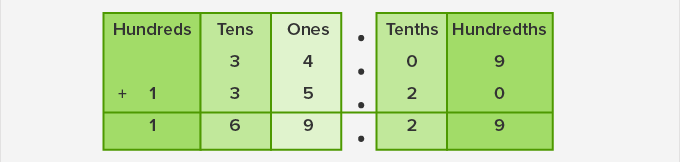Example: Subtract 119.05 from 250.47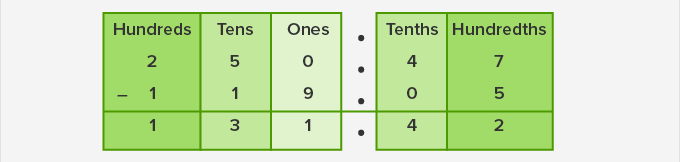Fun Facts The word decimal is derived from the Latin word “decimal” meaning a one-tenth part.

Won Numerous Awards & Honors